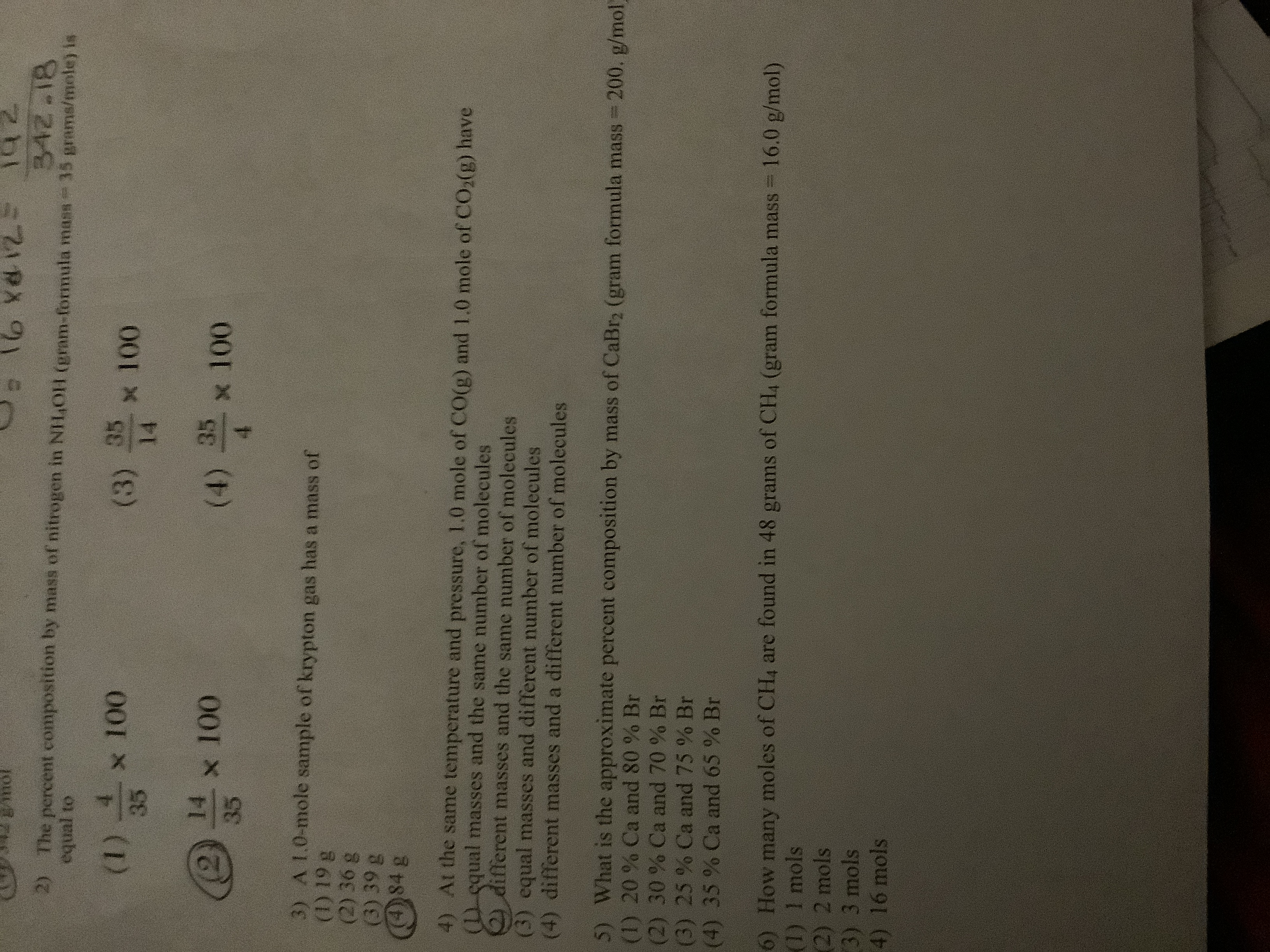# 1CE 342.18 35 grams/mole) is 2) The percent composition by mass of nitrogen in NI140H (gram-formula mass equal to (1) 4 x 100 (3) 35 x 100 35 14 x 100 35 x 100 (2 (4) 3) A I.0-mole sample of krypton gas has a mass of (1) 19 g (2) 36 g (3) 39 g 484 g 4) At the same temperature and pressure, 1.0 mole of CO(g) and 1.0 mole of CO2(g) have equal masses and the same number of molecules different masses and the same number of molecules (3) equal masses and different number of molecules (4) different masses and a different number of molecules 5) What is the approximate percent composition by mass of CaBr2 (gram formula mass = 200. g/mol) (1) 20 % Ca and 80 % Br (2) 30% Ca and 70 % Br (3) 25 % Ca and 75 % Br (4) 35 % Ca and 65 % Br moles of CH4 are found in 48 grams of CH4 (gram formula mass 6) How many (1) 1 mols (2) 2 mols 3) 3 mols 4) 16 mols 16.0 g/mol)

Question

question 4help_outlineImage Transcriptionclose1CE 342.18 35 grams/mole) is 2) The percent composition by mass of nitrogen in NI140H (gram-formula mass equal to (1) 4 x 100 (3) 35 x 100 35 14 x 100 35 x 100 (2 (4) 3) A I.0-mole sample of krypton gas has a mass of (1) 19 g (2) 36 g (3) 39 g 484 g 4) At the same temperature and pressure, 1.0 mole of CO(g) and 1.0 mole of CO2(g) have equal masses and the same number of molecules different masses and the same number of molecules (3) equal masses and different number of molecules (4) different masses and a different number of molecules 5) What is the approximate percent composition by mass of CaBr2 (gram formula mass = 200. g/mol) (1) 20 % Ca and 80 % Br (2) 30% Ca and 70 % Br (3) 25 % Ca and 75 % Br (4) 35 % Ca and 65 % Br moles of CH4 are found in 48 grams of CH4 (gram formula mass 6) How many (1) 1 mols (2) 2 mols 3) 3 mols 4) 16 mols 16.0 g/mol) fullscreen

### Want to see this answer and more?

Experts are waiting 24/7 to provide step-by-step solutions in as fast as 30 minutes!*